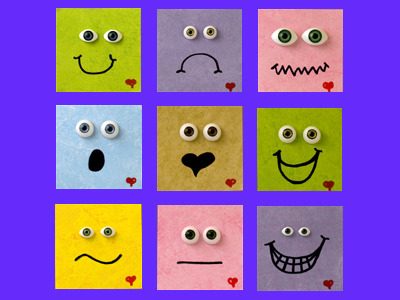Squares are one of the most common 2-D shapes. Triangles, circles and oblongs are also 2-D shapes.

# Year 2 Shapes - Describing 2D Shapes

This quiz addresses the requirements of the National Curriculum KS1 Maths and Numeracy for children aged 6 and 7 in year 2. Specifically this quiz is aimed at the section dealing with naming and describing 2D shapes and related everyday objects fluently.

When talking about 2-dimensional shapes and other related objects, Year 2 children will understand that they can use different words to describe their properties. They will use language such as sides, edges, corners and faces and recognise that some shapes have the same name but may look different.

This quiz will familiarise your child with the language used to describe 2-D shapes so that they can accurately name them and other related objects.

To see a larger image, click on the picture.
Question 1
What shape is this clock face?
A rectangle
A circle
A square
A triangle
All four sides are the same length
Question 2
How are rectangles different to squares?
They don't have as many corners
They have 4 sides of different lengths
They have two long and two short sides
They have more sides
Squares have 4 sides of equal length
Question 3
This triangle is called an equilateral triangle because all its sides and angles are..
different lengths
the same colour
the same
Equilateral means equal or the same
Question 4
This pattern is made up from rectangles. Which shape wouldn't fit together as well?
Triangles
Circles
Squares
Cubes
Because circles are curved, they don't fit together at all!
Question 5
A square is a kind of...
sphere
triangle
circle
rectangle
A rectangle has four sides - a square is a kind of rectangle with four equal sides
Question 6
This little girl is holding...
a triangle and a circle
a triangle and a square
a circle and a rectangle
a rectangle and a square
A triangle has three straight sides and a circle has one curved side
Question 7
Which shape has the most corners
They all have the same number of corners
The circle
The triangle
The square
The square has four corners, the triangle has three and the circle has no corners
Question 8
The coloured part of the eye is a...
rectangle
square
triangle
circle
The iris and pupil are both circles
Question 9
What shape are the pairs of triangles making?
Oblongs
Circles
Triangles
Squares
Two right-angled triangles pushed together can make a square
Question 10